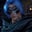Related Tags

communitycreator
python

# How to sort an array that contains 1 to n values in PythonGutha Vamsi Krishna

### Problem statement

You are given an array that contains 1 to n elements. Your task is to sort this array in an efficient way.

### Example

Input:[8,6,9,4,3,7,2,6,1]

Expected output: [1,2,3,4,5,6,7,8,9]

### Solution

We can solve this problem in two ways:

• Use the sort method.
• Use a for loop

### The sort() method approach

• The sort() method will sort the original list on which it is applied.
• Time complexity = $O(nlog \space n)$

In the code snippet below:

• We initialize list lst with [8,6,9,4,3,7,2,6,1].
• We print the original list lst.
• We use the lst.sort() method to sort the original list.
• Finally, we print the sorted list lst.
#initialize list
lst = [8,6,9,4,3,7,2,6,1]

#print original list
print("Original list  " + str(lst))

#sort the list
lst.sort()

#print the sorted list
print("Sorted list  " + str(lst))

### The for loop approach

Now, we will try to use a for loop to solve this in $O(n)$ time complexity.

### Approach

• Since the elements in the list are from 1 to n, we can fill the array one by one, like so:

• arr = 1, arr = 2,… arr[n-1] = n

To sort the list in the code below:

• We use a for in range loop and go until n-1, where n is the length of the list, which can be calculated with len(lst).
• We replace every element at i with i+1.
• After the loop completes, the list lst is filled with sorted numbers.
#initialize list
lst = [8,6,9,4,3,7,2,6,1]

#print original list
print("Original list  " + str(lst))

#sort the list
for i in range(len(lst)):
lst[i] = i+1

#print the sorted list
print("Sorted list  " + str(lst))

RELATED TAGS

communitycreator
python

CONTRIBUTORGutha Vamsi Krishna
RELATED COURSES

View all Courses

Keep Exploring

Learn in-demand tech skills in half the time